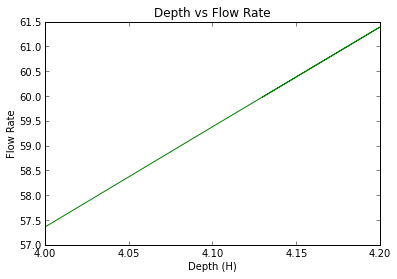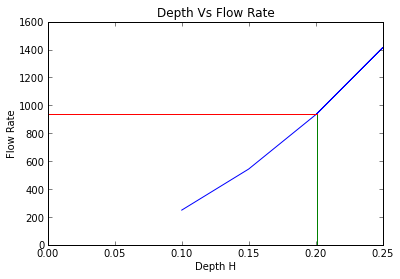# Chapter 7,Open channels notches and Weirs¶

## Example 7.2,Page 171¶

In :
#variable decleration
import math
l =1; # m
b =0.3; # m
n =0.014; # s /m^ ( 1 / 3 )
i =1.0/1000;

#calculations
A=l*b;
P =2* b+l;
m=A/P;
Q=A/n*m **(0.67) * math.sqrt (i);

#results
print"The delivery of water through the channel is found to be (m^3/s)= ",round(Q,3)

The delivery of water through the channel is found to be (m^3/s)=  0.221


## Example 7.3 Page 173¶

In :
%matplotlib inline
#variable decleration
import math
import numpy as np
from pylab import *
n =0.015; #m^(-1/3) s
i =1;
H =[4.0, 4.1, 4.2, 4.13];

#calculation
A =12* H;
P =12+2* H;
m=A/P;
C=m **(0.167) /n;
Q=C*A* math.sqrt (m*i);
#An analytical soln for depth H is not possible.It is so necessary to use a graphical soution
#The corresponding values of A, P, MHD (m) , Q are given below as taken from values of H

A =[48, 49.2, 50.4, 49.56];
P =[20, 20.2, 20.4, 20.26];
m =[2.4, 2.44, 2.47, 2.45];
Q =[57.36, 59.38, 61.39, 59.98];
figure()
plot (H,Q,'g')
r =[4.13, 4.13];
s =[57, 60];
plot (r,s, ' r ' )
t =[4, 4.13];
u =[60, 60];
plot (t,u, ' y ' )
xlabel('Depth (H)')
ylabel('Flow Rate')
title('Depth vs Flow Rate')
show()
# So, the depth is found to be approx. 4.13
depth =4.13; #m
C1 =(2.45) **(0.167) /n;

#results
print"Depth(m) = ",round(depth,3)
print"Chezy Coeff. =",round(C1,3)

Welcome to pylab, a matplotlib-based Python environment [backend: module://IPython.zmq.pylab.backend_inline].
For more information, type 'help(pylab)'.Depth(m) =  4.13
Chezy Coeff. = 77.428


## Example 7.4,Page 175¶

In :
#variable decleration
import math
Q =300.0/60; # m^3/ s
i =1.0/1600;

#calculation
H=(Q /140* math.sqrt (2/ i)) **(0.67) ;
A =2* H**2;

#result
print "The minimum flow area is found to be(m^2)= ",round(A,3)

The minimum flow area is found to be(m^2)=  5.132


## Example 7.5,Page 177¶

In :
%matplotlib inline
#variable decleration
from math import pi
import math
import numpy as np
from pylab import *
d =0.9144; # m
C =100; # m^ ( 1 / 2 ) s ^(-1)
R=d/2;
H =[0.1, 0.15, 0.2, 0.25, 0.201];

#calculations
theta = math.acos ((R-H)/R);
A=R **2*( theta -math.sin (2* theta )/2);
P =2* R* theta ;
m=A/P;
#An analytical soln for depth H is not possible.It is so necessary to use a graphical soution
#The corresponding values of A, P, MHD (m) , Q are given below as taken from values of H

theta =[0.674, 0.834, 0.973, 1.101, 0.975];
A =[0.039, 0.070, 0.106, 0.146, 0.107];
P =[0.616, 0.763, 0.890, 1.006, 0.891];
m =[0.063, 0.092, 0.119,0.145,0.120];
Q =[248.7, 543.2, 932.2 ,1412.9, 940.0];
figure()
plot (H,Q,)
xlabel('Depth H')
ylabel('Flow Rate')
title('Depth Vs Flow Rate');
i =[0.201, 0.201];
j =[0 ,940];
plot (i,j)
k =[0, 0.201];
l  =[940, 940];
plot (k,l)
show()
# So, the depth is found to be approx. 0.201
Depth =0.201; # m

#result
print"The depth in the channel(m) =",round(Depth,3)

Welcome to pylab, a matplotlib-based Python environment [backend: module://IPython.zmq.pylab.backend_inline].
For more information, type 'help(pylab)'.The depth in the channel(m) = 0.201


## Example 7.7,Page 180¶

In :
#variable decleration
import math
Cd =0.56;
B =1.2; # m
g =9.8; # m/ s ^2
H =0.018; # m

#calcualtion
Q =2.0/3* Cd*B* math.sqrt (2*g)*H **(1.5) ;

#results
print "The rate of flow of liquid over the weir is (m^3/s)=",round(Q,4)

The rate of flow of liquid over the weir is (m^3/s)= 0.0048


## Example 7.8,Page 182¶

In :
#variable decleration
from math import pi
import math
H2 =5.5;
Q1 =217.0;
Q2 =34.0;
H1 =8.5;

#calculation
H0 =( H2 *( Q1/Q2) **(0.67) -H1)/(( Q1/Q2) **(0.67) -1);

#result
print"The height of the weir crest above the surface of the river is found to be(m)",round(H0,2)

The height of the weir crest above the surface of the river is found to be(m) 4.28


## Example 7.9,Page 184¶

In :
#variable decleration
from math import pi
from scipy import integrate
import math
H =0.07; # a v e r a g e head
rate = -0.02/600; # (dH/ dt )
H1 =0.08; # m
H2 =0.01; # m

#calculation
k=- rate /H **(1.5) ;
def integrand(H,k):
return -1/k*H**(-1.5);

t=integrate.quad(integrand,H1,H2,args=(k))

#result
print "Time taken (s) =",round(t,2);

Time taken (s) = 7183415.25


## Example 7.10,Page 186¶

In :
#variable decleration
import math
Cd =0.62;
g =9.81; # m/ s ^2
H =0.03; # m

#calculations
Q =8.0/15* Cd* math.sqrt (2*g)*H **(2.5) ;

#results
print"Rate of flow (m^3/s) =",round(Q,6)

Rate of flow (m^3/s) = 0.000228


## Example 7.11,Page 188¶

In :
from scipy import integrate
#variable decleration
import math
l =4; # m
b =2; # m
H1 =0.15; # m
H2 =0.05; # m
A=8;

#calculation
k=3*1.5/A;
def integrand (H,A):
return -A/1.5*H**(-2.5)

t=integrate.quad(integrand,H1,H2,args=(A))

#results
print "Time taken to reduce the head in the the tank (s)=",round(t)

Time taken to reduce the head in the the tank (s)= 257.0

In [ ]: